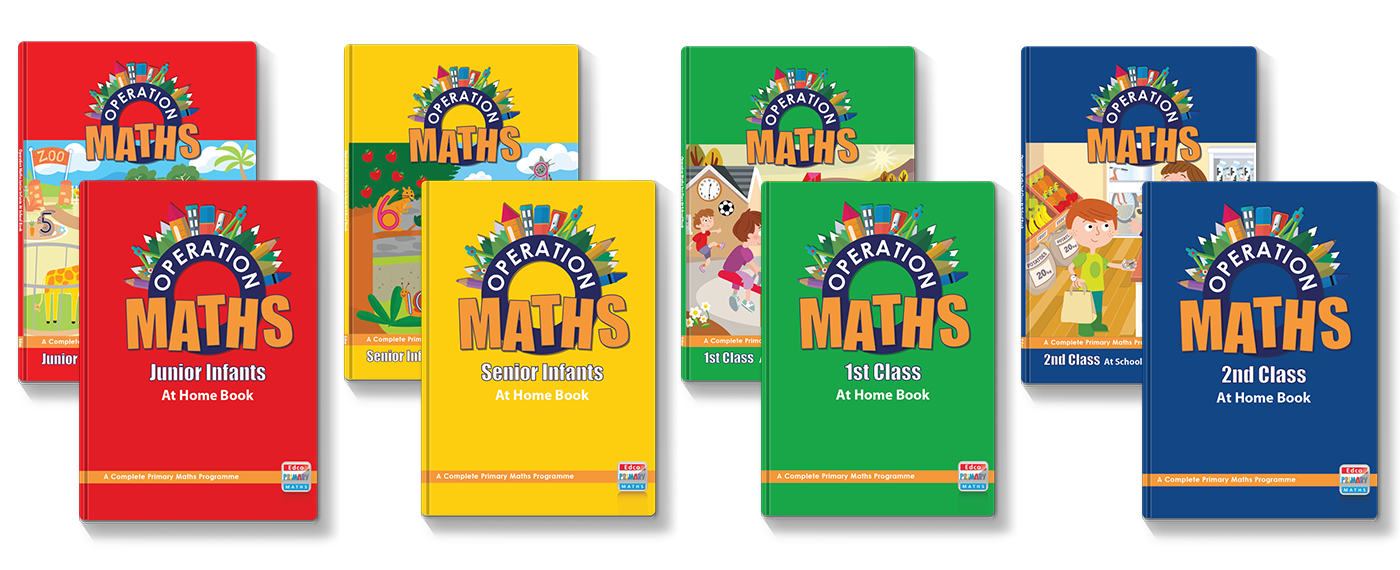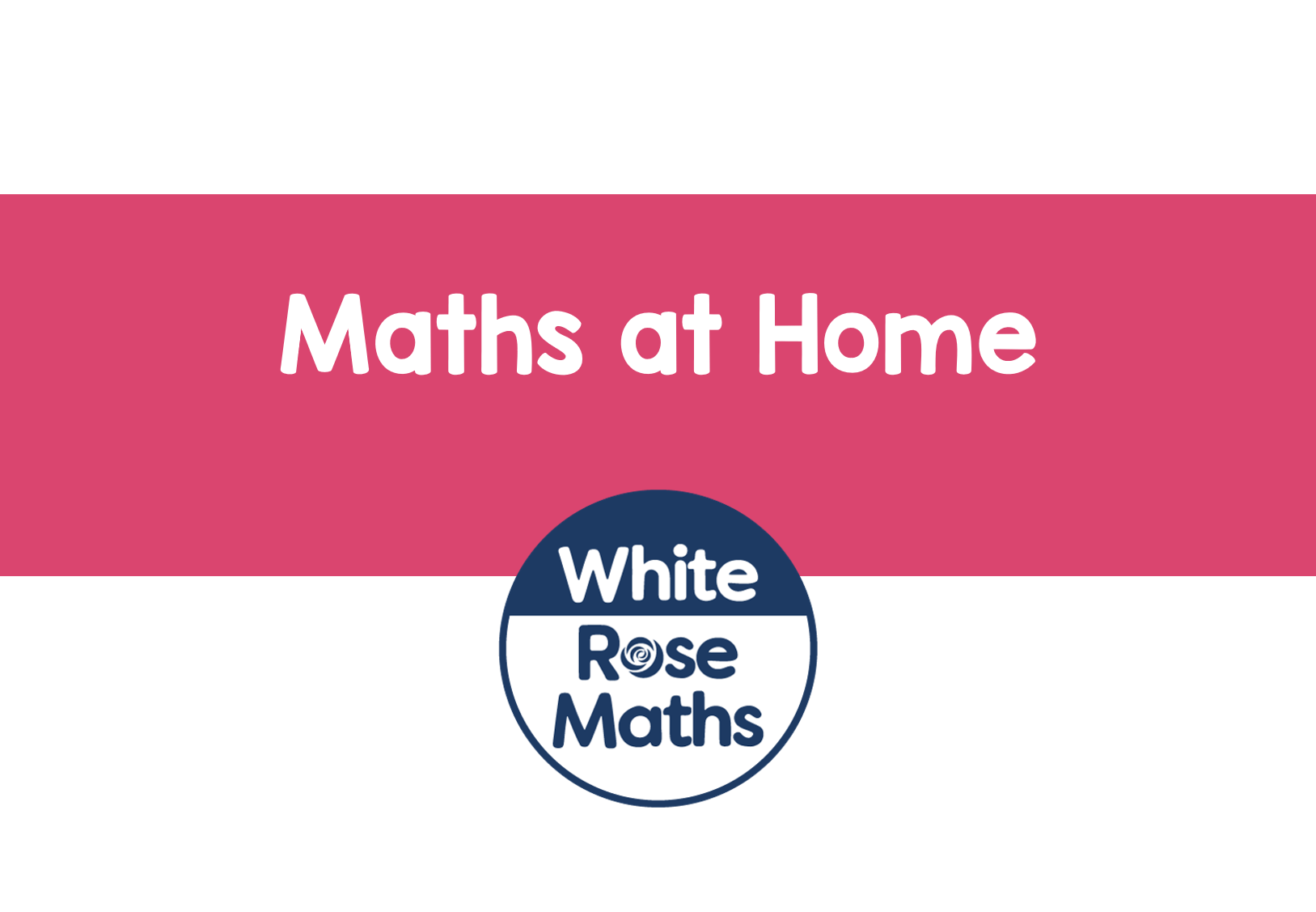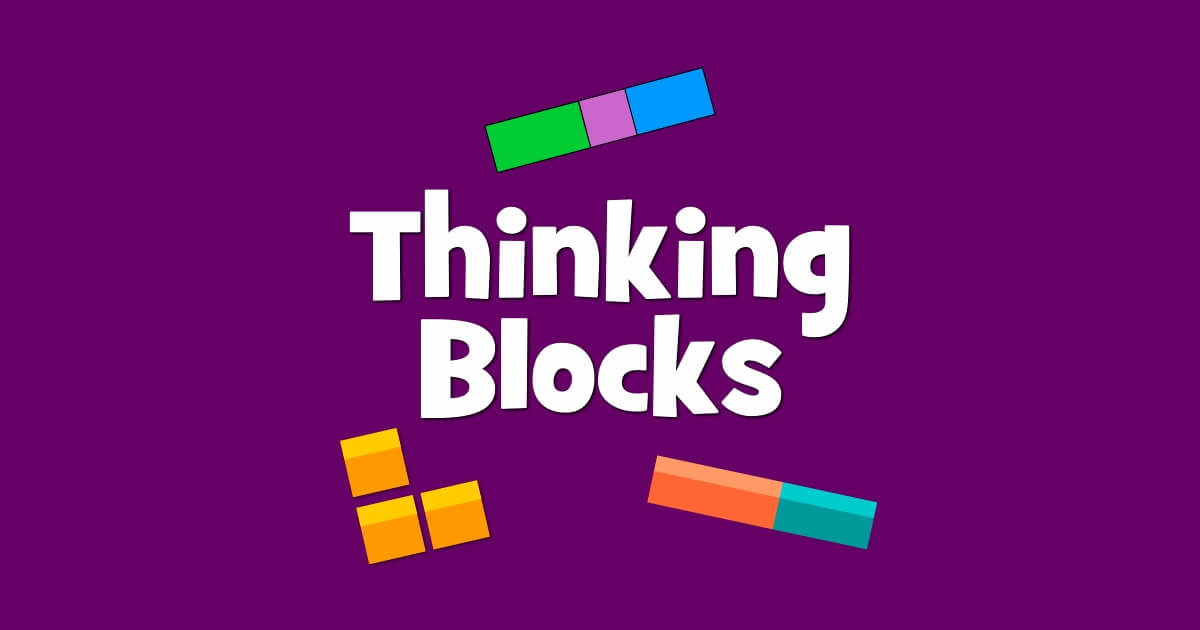# Dear Family, your Operation Maths guide to Fractions

## Dear Family, your Operation Maths guide to Fractions

Category : Uncategorized

Dear Family, given below is a brief guide to understanding the topic of fractions, as well as some practical suggestions as to how you might support your children’s understanding at home. Also below, are a series of links to digital resources that will help both the children, and you, learn more about fractions. The digital resources are organised according to approximate class level:You can also find class specific tips at the back of your child’s Operation Maths At Home book, for infants to second class, and in the Operation Maths Dear Family letters for third to sixth class.

#### Understanding fractions

Fraction comes from a Latin word meaning “to break (something) in pieces”; it’s related to the word fracture, meaning to break into pieces, usually used to describe a broken bone. In maths, fractions are the result of dividing something (a shape, object or amount), into a number of equal pieces or parts.

Equal is very important; if we divide something into two parts, but they are not equal, then they are not halves. Therefore it is very important to emphasise to children from the very beginning that the parts made must be equal; in school we often talk about it being a fair share.

And while fractions might seem straight-forward enough, i.e. dividing something into equal parts, even the basic fractions can be interpreted in many ways:

3/4 (say “three quarters”) can be describing 3 parts of something that is is divided into 4 equal parts, eg 3/4 of the square is red (above) or 3/4 of the blocks are red (below).

3/4 can also be interpreted as three out of four i.e. that 3 out of 4 cubes are red or that 3 out of every group of 4 cubes are red (below).

3/4 can also be interpreted as three divided by four or 3 ÷ 4 which gives a value of 0.75, the decimal equivalent for 3/4 (below).

Initially much of children’s exploration of fractions in school, will focus on creating and identifying various fractions (starting with familiar fractions such halves, quarters, eighths, tenths etc).

They will also learn to recognise fraction forms that are the same value (i.e. equivalent fractions eg 1/2, 2/4, 4/8, 5/10 etc), and comparing and ordering fractions. In the senior classes, children will work with less familiar, more complex fractions as well as calculating with, adding, subtracting, multiplying and dividing fractions.

#### Practical Suggestions for Supporting Children

• Use fractions and the language of fractions at home, e.g. when dividing up food (cutting up pizzas, cake, bars of chocolate), when baking (e.g. half a teaspoon, a quarter of a cup, half a dozen eggs).
• Look for fractions when out shopping, e.g. half price. Ask your child to calculate the current price, or what the price was before the reduction.
• Be careful about using fractional language accurately, e.g. there’s no such thing as a big half or a small half! To make fractions, we must create equal parts. Encourage your child to be accurate and to recognise that if something is divided into two parts that are not equal, they are not halves.
• Fractions names can be confusing for children: it makes sense that each part of something divided into ten equal parts is a tenth… similarly for fifth, sixth, eighth, ninth etc. But each part of something divided into two equal parts is a half (plural is halves) and each part of something divided into four equal parts is a quarter. Furthermore, when speaking one eighth (1/8) it can be difficult to distinguish from eight (8). Try to say and explain fraction names clearly, and encourage your child to say them clearly also, so that they appreciate the difference between these similar-sounding words.
• If your child is experiencing difficulties with converting fractions, adding, subtracting or multiplying fractions, encourage him/her to use concrete materials (eg paper plates, paper straws and or pieces of paper) and/or draw pictorial representations, e.g. pie pieces or number lines, to help them visualise what is happening and develop his/her “fraction sense”.

#### Digital Resources for First and Second ClassesKhan Academy Fractions: Watch the videos and then answer the practice questions on halves and fourths (another name for quarters). Afterwards, try the activities in Second Grade. You can also register for a free Khan Academy account to record your progress and explore other areas of First Grade Maths.Hit the Button: Use the Halves game to practice calculating half of a numberFractions practice games from ixl.com. You can do a number of free quizzes each day without having a subscription. (Please note that the class levels given do not always align accurately with the content of the Irish Primary Curriculum)

#### Digital Resources for Third and Fourth ClassesHappy Numbers Third Grade: Third and fourth class pupils could work through the activities in Module 5.White Rose Fractions: a series of lessons on Fractions for year 3 and year 4.Khan Academy Fractions (Third Grade): Watch the videos and then answer the practice questions. Afterwards, try Equivalent Fractions, and for more challenging,  look at the Fourth Grade Activities. You can also register for a free Khan Academy account to record your progress and explore other topics/grades.Fractions Intro: An interactive fractions simulation which explores fractions, equivalent fractions and improper fractions. The accompanying fractions game has various levels of difficulty. Other related fractions simulations at this site include Build a Fraction, Fraction Matcher, Equivalent Fractions and Mixed Numbers.That Quiz – Fractions: Use this quiz to practice Identifying Fractions and Comparing Fractions. You can choose different options from the menu on the left-hand side.I Know it! – Fractions (Third Grade): Scroll down to the Fractions & Decimals section to do any of the activities. You could also try the Fractions sections in Fourth Grade.Hit the Button: Use the Halves game to practice calculating half of a numberBattleship Numberline: Can you blow up the enemy submarines? This game starts very easy, where you must click the correct fraction on the number line, but then the game progresses in difficulty as the player must work out where a given fraction would be placed on the blank number line. Choose the fractions game.Splash Learn – Fraction Games: An assortment of games organised according to US grade levels; start with the games for third grade level. If too simple, go to the Grade Four games.Fractions practice games from ixl.com. You can do a number of free quizzes each day without having a subscription. (Please note that the class levels given do not always align accurately with the content of the Irish Primary Curriculum)

#### Digital Resources for Fifth and Sixth ClassesHappy Numbers Fourth Grade: Pupils should start with the activities in Module 3. And then progress to Fifth Grade to do the activities in Module 3 and 4White Rose Fractions: a series of lessons on fractions. These lessons could be followed up with other fraction lessons in year 5 and/or year 6.Khan Academy Fractions: Learn how to Add and Subtract Fractions and Multiply Fractions. Afterwards, for more of a challenge,  look at more on Add & Subtract Fractions, Multiply Fractions and Divide Fractions. You can also register for a free Khan Academy account to record your progress and explore other topics/grades.Fractions Intro: An interactive fractions simulation which explores fractions, equivalent fractions and improper fractions. The accompanying fractions game has various levels of difficulty. Other related fractions simulations at this site include Build a Fraction, Fraction Matcher, Equivalent Fractions and Mixed Numbers.Thinking Blocks for Fractions: an ideal way to start using bar models, or further practice using bar models, to model fraction word problems and computation. Sixth class pupils could also try out the thinking blocks activities on ratio.That Quiz – Fractions: Use this quiz to practice Identifying FractionsComparing Fractions and Operations with Fractions. You can choose different options from the menu on the left-hand side.I Know it! – Fractions (Fourth Grade): Scroll down to the Fractions & Decimals section to do any of the activities. You could also try the Fractions sections in Fifth Grade.Hit the Button: Use the Halves game to practice calculating half of a numberBattleship Numberline: Can you blow up the enemy submarines? This game starts very easy, where you must click the correct fraction on the number line, but then the game progresses in difficulty as the player must work out where a given fraction would be placed on the blank number line. Choose the fractions game.Splash Learn – Fractions: An assortment of place value games organised according to US grade levels; start with the grade below your current class level i.e. for fifth class pupil’s start with fourth grade games and for sixth class pupils start with fifth grade games.Fractions practice games from ixl.com. You can do a number of free quizzes each day without having a subscription. (Please note that the class levels given do not always align accurately with the content of the Irish Primary Curriculum)